# Average speed

What is the average speed you have to move the way around the world in 80 days? (Path along the equator, round to km/h).

v =  21 km/h

### Step-by-step explanation: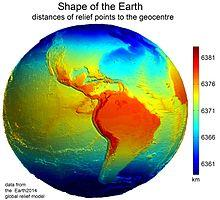We will be pleased if You send us any improvements to this math problem. Thank you!Tips to related online calculators
Do you want to convert length units?
Do you want to convert velocity (speed) units?
Do you want to round the number?

#### You need to know the following knowledge to solve this word math problem:

We encourage you to watch this tutorial video on this math problem:

## Related math problems and questions:

• Earth's diameter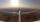The Earth's diameter on the equator is approximately 12750 km. How long does the Gripen flyover the Earth above the equator at 10 km if it is at an average speed of 1500 km / h?
• Sputnik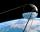The first Earth satellite was flying at speed 8000 m/s. At that rate he circled the earth in 82 minutes. Jet flies at an average speed 800 km/h. How long would it take circle the earth round?
• Earth rotation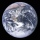How fast is the place on the Earth's equator moving if the Earth's radius is 6378 km?
• Average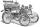What is the average speed of the car, where half of the distance covered passed at speed 66 km/h and the other half at 86 km/h.
• Cars motion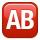From point A car start at the speed of 90 km/h. Against it departs from the point B at the same time another car at 60 km/h. For how long and where they met if the distance between A and B is 180 km?
• Where and when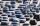The truck left Kremnica at 11:00 h at a speed of 60km/h. At 12:30 h, the passenger car started at an average speed of 80km/h. How many kilometers from Kremnica will the passenger car reach truck, and when?
• Motorcyclist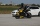From Trutnov, the motorcyclist started at an average speed of 60km/hour. At 12.30hrs the passenger car was started at a speed of 80km/hour. How many hours and at what distance from Trutnov will car catch a motorcycle?
• MotionFrom two different locations distant 232 km started against car and bus. The car started at 5:20 with average speed 64 km/h. Bus started at 7:30 with average speed 80 km/h. When they meet? How many kilometers went the bus?
• Constant motionThe car has undergone constant motion 120 km in 1 hour 30 minutes. Determine its average speed. Determine how long car drove in the first 20 minutes and 50 minutes from the start of the move.
• The equatorCalculate the length of the equator. Calculate a radius of 6378 km. Round to the nearest thousand. - Procedure and calculation
• Average speedThe car drove from city A to city B at a 40 km/h and back to city A at a speed of 80 km/h. What was the average speed of the car?
• Athletic competitionIn a 400 meter athletic competition, a participant covers the distance as given below. find the average speed? first 80 meters 10 m/s next 240 meters 7.5 m/s last 80 meters 10 m/s
• Average speedThe truck drove 1/2 of the way on the highway at 80km/h. The other half of the way 20km/h. Calculate the average speed
• CarThe driver of a car is to get to 608 km distant city. From atlas found that 162 km will have to pass through the cities at average speed 48 km/h. Remainder of the journey pass outside the cities at average speed 116 km/h. Calculate how many hours it will
• Average speed 4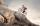Starting at home, Tony traveled uphill to the store for 45 minutes at 8 miles per hour. he then traveled back home on the same path at a speed of 24 miles per hour. What is his average speed for the entire trip?
• Two airportsTwo airports are 2690 km away. From the first airport, the airplane flies at a speed of 600 km/h, from a second airplane at a speed of 780 km/h. When will they meet if they flew at 10:00? How far from the first airport?
• Two citiesCities A and B are 200 km away. At 7 o'clock from city A, the car started at an average speed of 80 km/h, and from B at 45 min later the motorcycle is started at an average speed of 120 km/h. How long will they meet and at what distance from the point A i